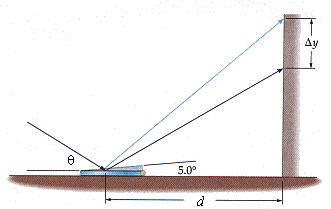# Ray Optics Question

Here is the problem I am working on:

Sunlight enters a room at an angle of theta = 32° above the horizontal and reflects from a small mirror lying flat on the floor. The reflected light forms a spot on a wall that is d = 1.9 m behind the mirror, as shown in Figure 26-42. If you now place a pencil under the edge of the mirror nearer the wall, tilting it upward by 5.0°, how much higher on the wall (delta y) is the spot?Here is what I have done:

delta y = yf - yi = d tan thetaf - d tan thetai = (1.9m)(tan37 - tan32) = 24.45 cm

In WebAssign, I submitted both 24.45 cm and 24 cm, but neither was accepted. What am I doing wrong? My instructor said my answer is not close to that in WebAssign.

Doc Al
Mentor
FlipStyle1308 said:
Here is what I have done:

delta y = yf - yi = d tan thetaf - d tan thetai = (1.9m)(tan37 - tan32) = 24.45 cm

In WebAssign, I submitted both 24.45 cm and 24 cm, but neither was accepted. What am I doing wrong? My instructor said my answer is not close to that in WebAssign.
You have the wrong angle. When the mirror's flat, the normal line to its surface points straight up. But when it's tilted, where does the normal line point? Now apply the law of reflection about that new normal.

George Jones
Staff Emeritus
Gold Member
Draw a separate diagram for the second situation, and put in the angles carefully.

Edit: Doc beat me to it.

Doc Al said:
You have the wrong angle. When the mirror's flat, the normal line to its surface points straight up. But when it's tilted, where does the normal line point? Now apply the law of reflection about that new normal.

When the mirror's tilted, the normal line points upward, away from the wall, right? So does this mean the angle I should replace is supposed to be 85 degrees?

OlderDan
Homework Helper
FlipStyle1308 said:
When the mirror's tilted, the normal line points upward, away from the wall, right? So does this mean the angle I should replace is supposed to be 85 degrees?
When you tilt the mirror, the angle θ changes, so the reflection angle changes. But these angles are relative to the plane of the mirror, and the mirror is no longer flat on the table.

OlderDan said:
When you tilt the mirror, the angle θ changes, so the reflection angle changes. But these angles are relative to the plane of the mirror, and the mirror is no longer flat on the table.

Okay, so I drew myself a picture. Originally the normal line is straight up, as Doc Al said. As the mirror is tilted 5 degrees, the normal line is now pointing 5 degrees in the upper left direction. Theta changes and increases to 37 degrees. But on the other side of the normal line, theta is 42 degrees, is that right? So I replace 37 with 42, or am I still doing something wrong?

OlderDan